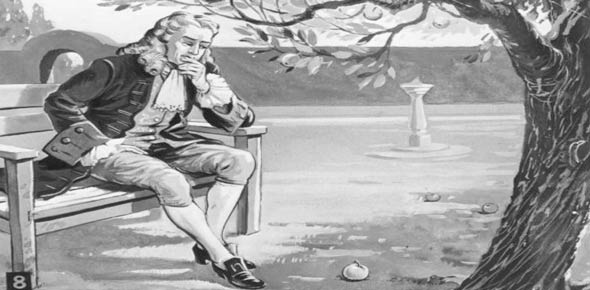# Law Of Motion

5 Questions | Total Attempts: 266SettingsNewtons Law

Related Topics
• 1.
What is inertia?
• A.

The tendency of an object to continue doing what it is currently doing.

• B.

The opposing force of gravity.

• C.

The mass of an object.

• D.

Another name for unbalanced forces.

• 2.
How long will an object remain at rest, according to Newton's First Law of Motion?
• A.

It can't rest.

• B.

Until noon.

• C.

As long as there are unbalanced forces acting on it.

• D.

Until an unbalanced force occurs.

• 3.
How can you tell if an object is in motion?
• A.

The object will look different.

• B.

I'll be able to see the forces.

• C.

It will remain in the same position.

• D.

It's position will change in relation to other objects.

• 4.
Which of Newton's Laws describes the amount of force applied to accelerate an object's mass?
• A.

Newton's First Law

• B.

Newton's Second Law

• C.

Newton's Fourth Law

• D.

Newton's Third Law

• 5.
For every ___ there is an equal and opposite ______.
• A.

Reaction, action

• B.

Action, action

• C.

Action, reaction

• D.

Reaction, reaction